# Cover Image from A New Kind of Science

The cellular automaton evolution shown on the cover of Stephen Wolfram’s A New Kind of Science

This includes the initial conditions and raw data for the cellular automaton evolution, as given on page 851.

## Examples

### Basic Examples

Retrieve the data:

 In:=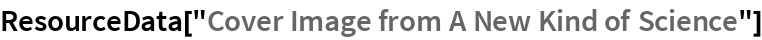Out=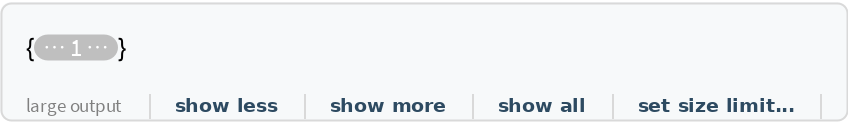Show in the colors used on the book:

 In:=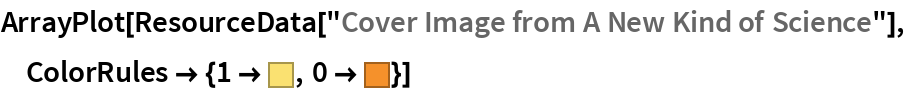Out=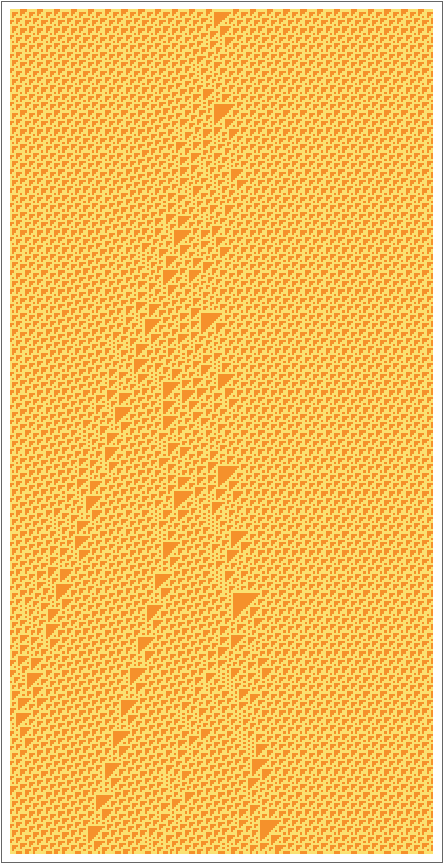Find the initial condition for the cellular automaton:

 In:=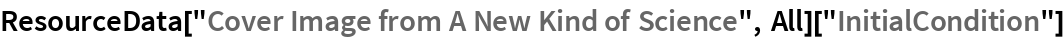Out=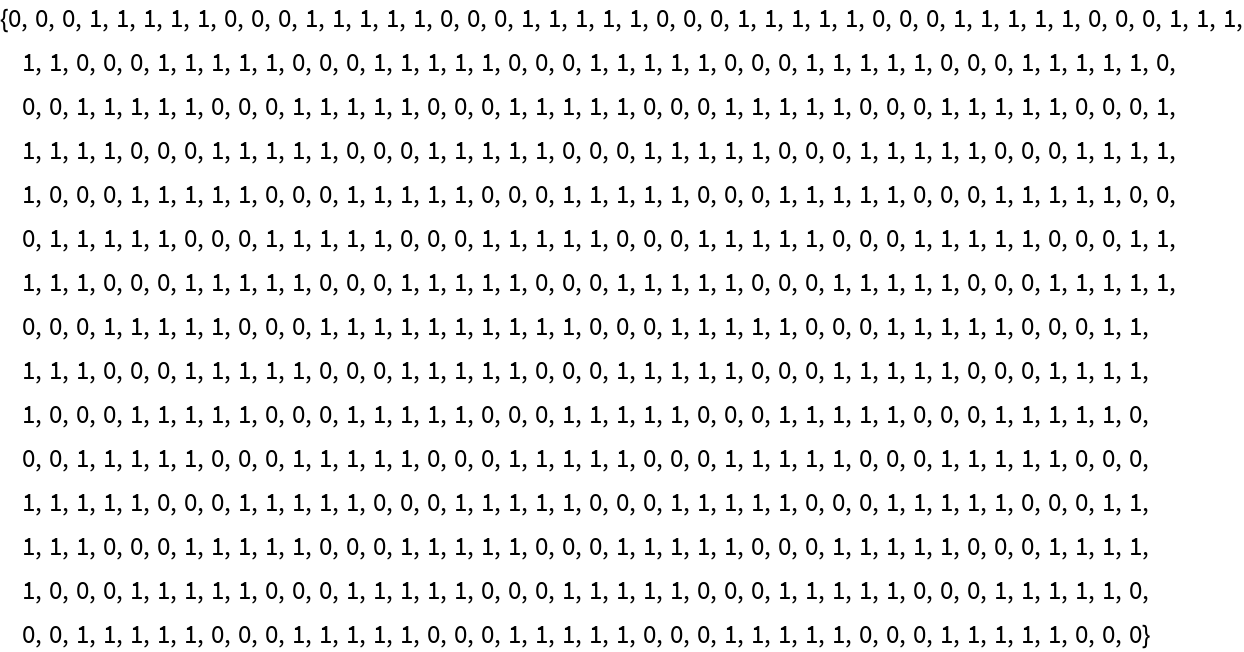In:=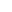Wolfram Research, "Cover Image from A New Kind of Science" from the Wolfram Data Repository (2017) https://doi.org/10.24097/wolfram.50863.data